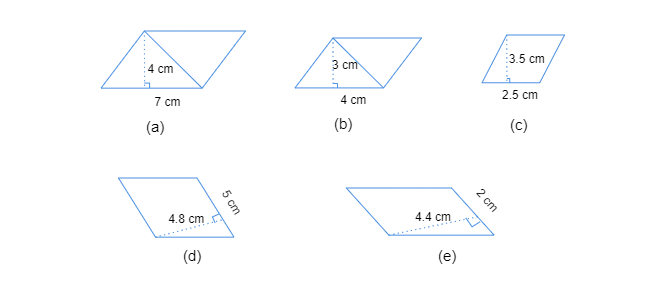# Find the area of each of the following parallelograms:"

To do: To find the area of each of the given parallelograms.

Solution:

$(a)$. Area of the parallelogram

$=base\times altitude$

$=7\ cm\times4\ cm$

$=28\ cm^2$

$(b)$. Area of the parallelogram

$=base\times altitude$

$=5\ cm\times3\ cm$

$=15\ cm^2$

$(c)$. Area of the parallelogram

$=base\times altitude$

$=2.5\ cm\times3.5\ cm$

$=8.75\ cm^2$

$(d)$. Area of the parallelogram

$=base\times altitude$

$=5\ cm\times4.8\ cm$

$=24\ cm^2$

$(e)$. Area of the parallelogram

$=base\times altitude$

$=2\ cm\times4.4\ cm$

$=8.8\ cm^2$